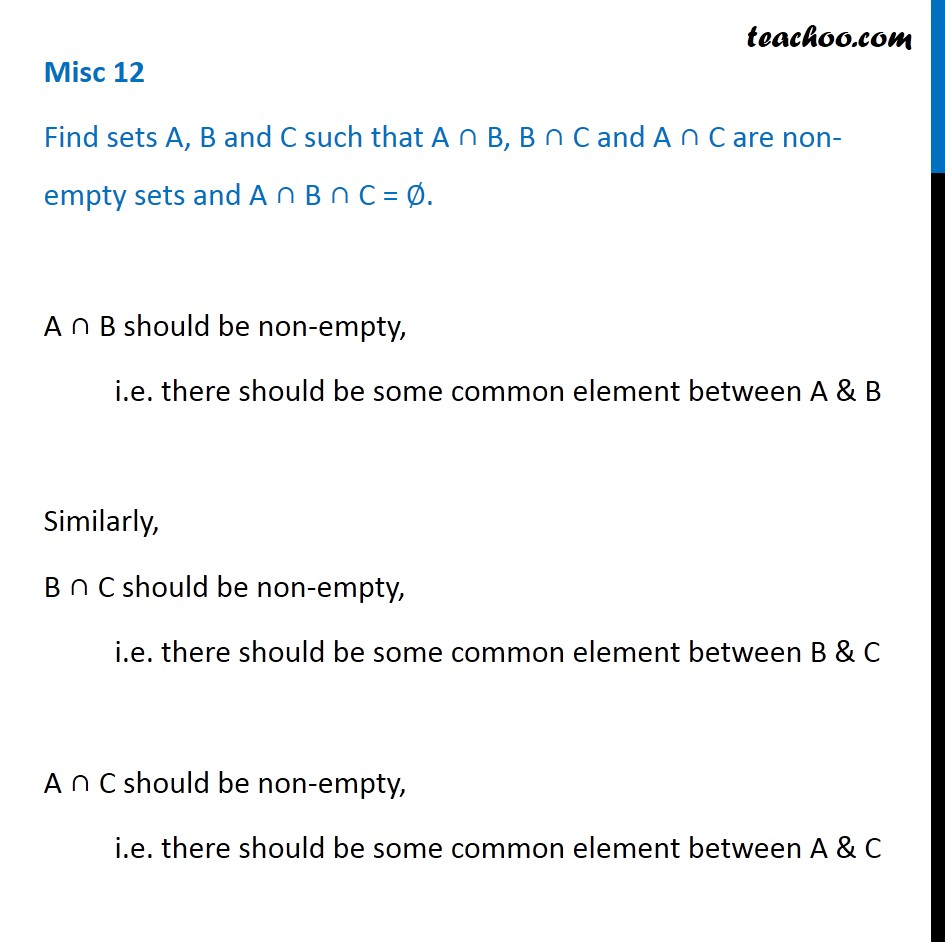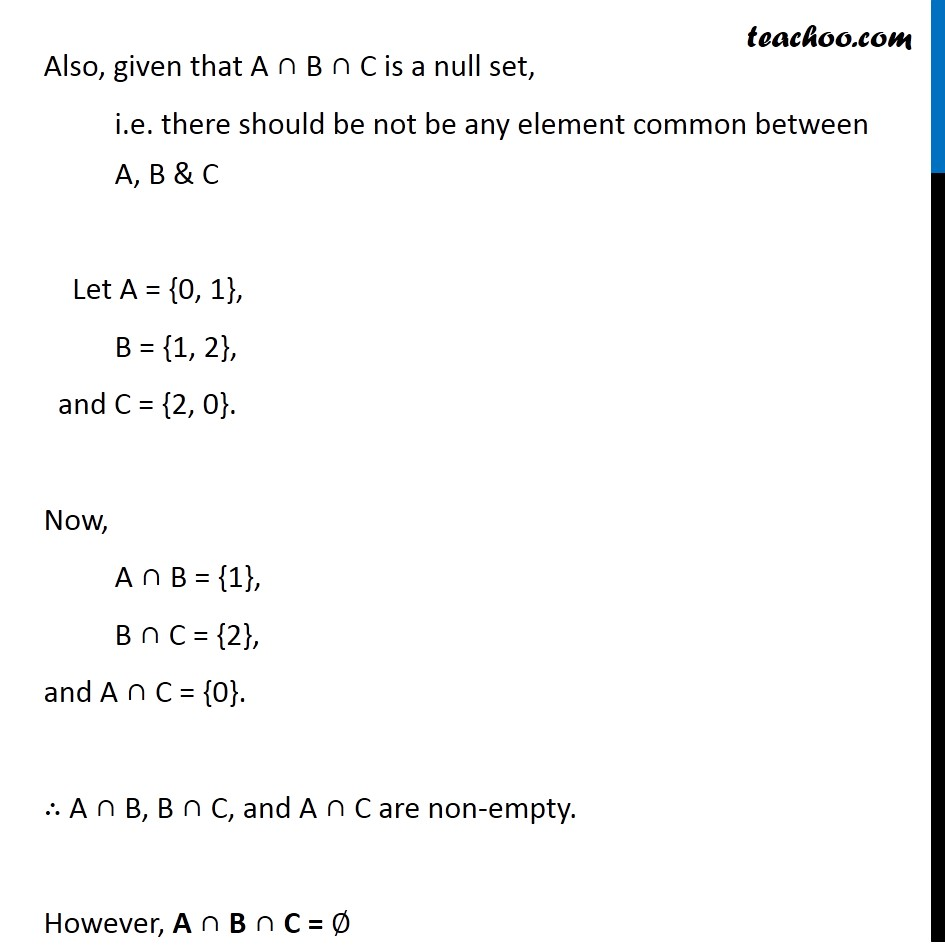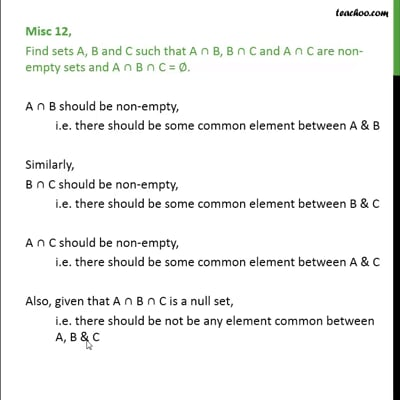Proof - Using properties of sets

Chapter 1 Class 11 Sets
Concept wiseThis video is only available for Teachoo black users

Introducing your new favourite teacher - Teachoo Black, at only ₹83 per month

### Transcript

Misc 12 Find sets A, B and C such that A ∩ B, B ∩ C and A ∩ C are non-empty sets and A ∩ B ∩ C = ∅. A ∩ B should be non-empty, i.e. there should be some common element between A & B Similarly, B ∩ C should be non-empty, i.e. there should be some common element between B & C A ∩ C should be non-empty, i.e. there should be some common element between A & C Also, given that A ∩ B ∩ C is a null set, i.e. there should be not be any element common between A, B & C Let A = {0, 1}, B = {1, 2}, and C = {2, 0}. Now, A ∩ B = {1}, B ∩ C = {2}, and A ∩ C = {0}. ∴ A ∩ B, B ∩ C, and A ∩ C are non-empty. However, A ∩ B ∩ C = ∅